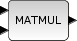Change language to:
English - Français - 日本語 - Português -

See the recommended documentation of this function

# MATMUL

Matrix Multiplication

### Block Screenshot### Description

The MATMUL block computes the multiplication of an the first input matrix by the second input matrix/scalar. When the Multiplication rule parameter is set to:

• 1, the block computes the matrix product. The number of rows of the first matrix must be equal to the number of columns of the second matrix. The output is a matrix where the number of rows is equal to that of the first input matrix and the number of columns is equal to that of the second input matrix.

• 2, the block computes the matrix element-wise product. The matrices must be of the same sizes. The output is a matrix of the same size that the input matrices.

• 3, the block computes the matrix-scalar product. The output is a matrix of the same size that input matrix.

For integer inputs, when overflow occurs the block three different forms of results :

1- A normal non saturated result. By example, if type is int8 and the result 128, the block output value will be -128.

2- A saturated result. For the previous example the block output value will be 127.

3- An error message warning the user about the overflow.

The user can select one of these three forms by setting the Do on Overflow field to 0, 1 or 2.

### Dialog box• Datatype(1=real double 2=Complex)

It indicates the type of the output. It support only the two types double (1) and complex (2). If we input another entry in this label xcos will print the message "Datatype is not supported".

Properties : Type 'vec' of size 1.

• Multiplication rule (1= * 2= .* 3=scalar)

Properties : Type 'vec' of size 1.

• Do on Overflow(0=Nothing 1=Saturate 2=Error)

When this parameter is set to zero the result is similar to a normal multiplication of two integer matrix. When it is set to 1, on overflow the block saturate the result. When it is set to 2, on overflow an error message box appears.

For double or complex inputs this parameter is ignored.

Properties : Type 'vec' of size 1.

### Example

```A=[1 2 3;4 5 6]
B=[9 8 7 6;5 4 3 2;9 7 5 3]
y=[46 37 28 19;115 94 73 52]```

### Default properties

• always active: no

• direct-feedthrough: yes

• zero-crossing: no

• mode: no

• regular inputs:

- port 1 : size [-1,-2] / type 1

- port 2 : size [-2,-3] / type 1

• regular outputs:

- port 1 : size [-1,-3] / type 1

• number/sizes of activation inputs: 0

• number/sizes of activation outputs: 0

• continuous-time state: no

• discrete-time state: no

• object discrete-time state: no

• name of computational function: matmul_m

### Interfacing function

• SCI/modules/scicos_blocks/macros/MatrixOp/MATMUL.sci

### Computational function

• SCI/modules/scicos_blocks/src/c/matmul_m.c

• SCI/modules/scicos_blocks/src/c/matzmul_m.c

• SCI/modules/scicos_blocks/src/c/matmul2_m.c

• SCI/modules/scicos_blocks/src/c/matzmul2_m.c